maths > mensuration-basics

Area of a Triangle

what you'll learn...

Overview

Area of a Triangle:
$\frac{1}{2}×\phantom{\rule{1ex}{0ex}}\text{base}\phantom{\rule{1ex}{0ex}}×\left(height\right)$$\frac{1}{2} \times \textrm{b a s e} \times \left(h e i g h t\right)$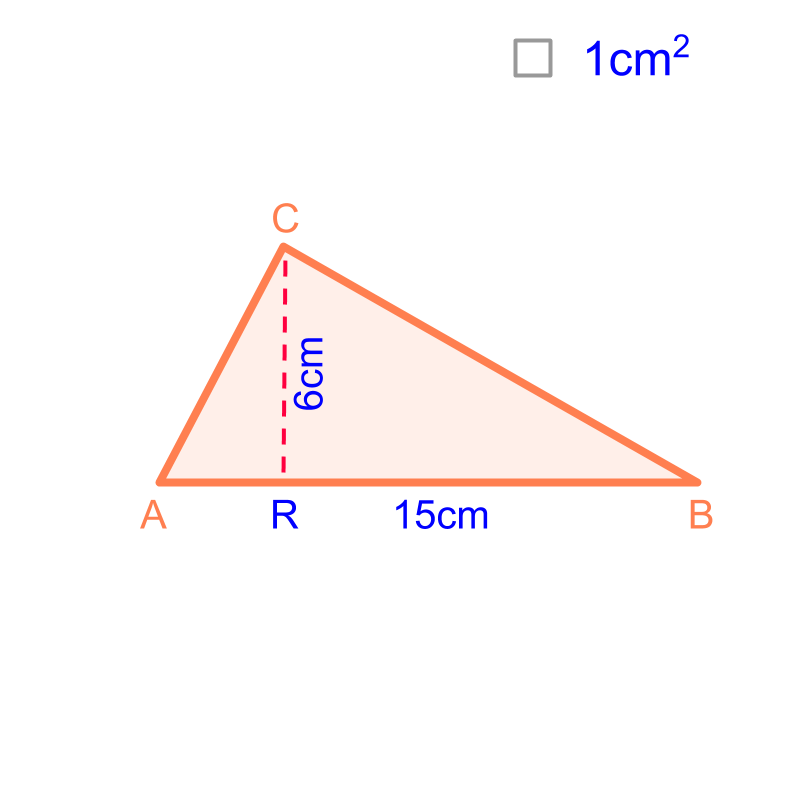type 1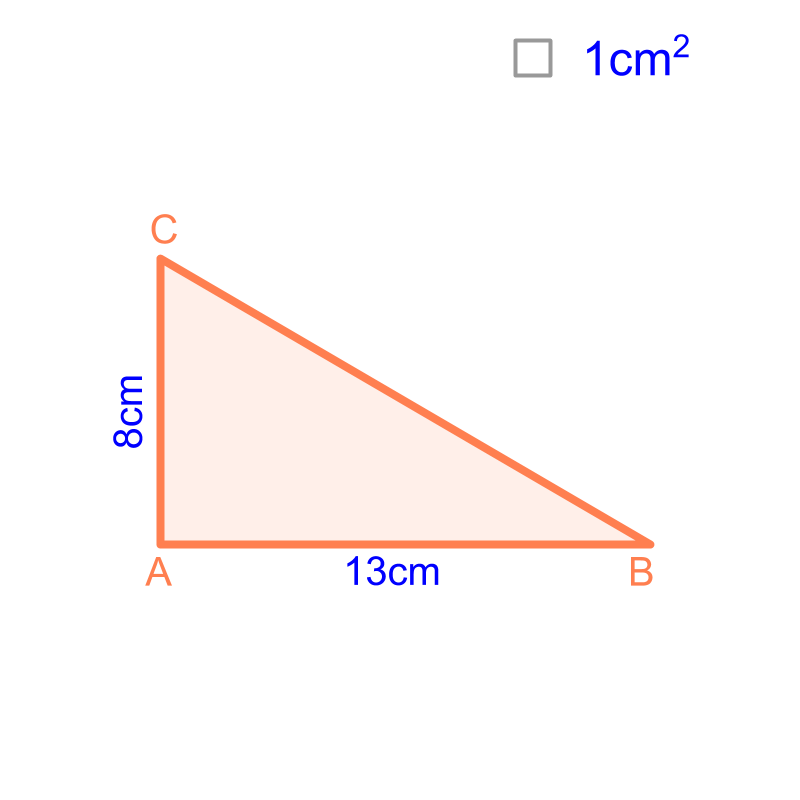Consider the triangle given in the figure. The base is $13$$13$ cm and height is $8$$8$ cm. To find the area of the triangle, compare the triangle with a rectangle of $13$$13$ cm by $8$$8$ cm.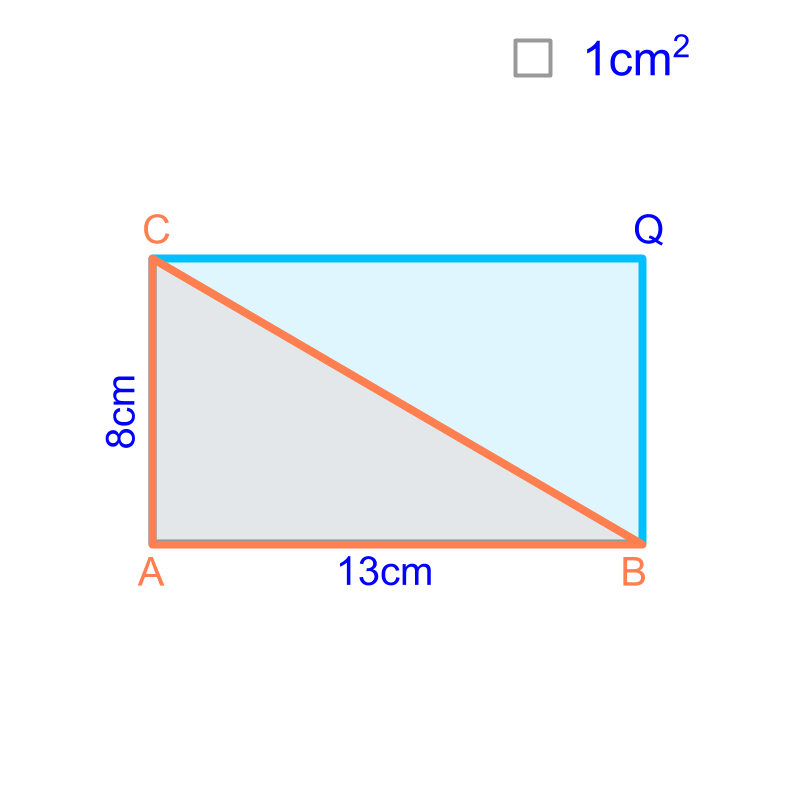The triangle of base $13$$13$ cm and height $8$$8$ cm is placed over a rectangle of length $13$$13$ cm and width $8$$8$ cm. It is noted that the triangle splits the rectangle into equal halves. The area of the triangle is half of the area of rectangle OR in other words, $\frac{1}{2}×\phantom{\rule{1ex}{0ex}}\text{base}\phantom{\rule{1ex}{0ex}}×\left(height\right)$$\frac{1}{2} \times \textrm{b a s e} \times \left(h e i g h t\right)$

type 2Consider the triangle given in the figure. The base is $15$$15$ cm and height is $6$$6$ cm. To calculate the area, compare the triangle with a rectangle of $15$$15$ cm by $6$$6$ cm.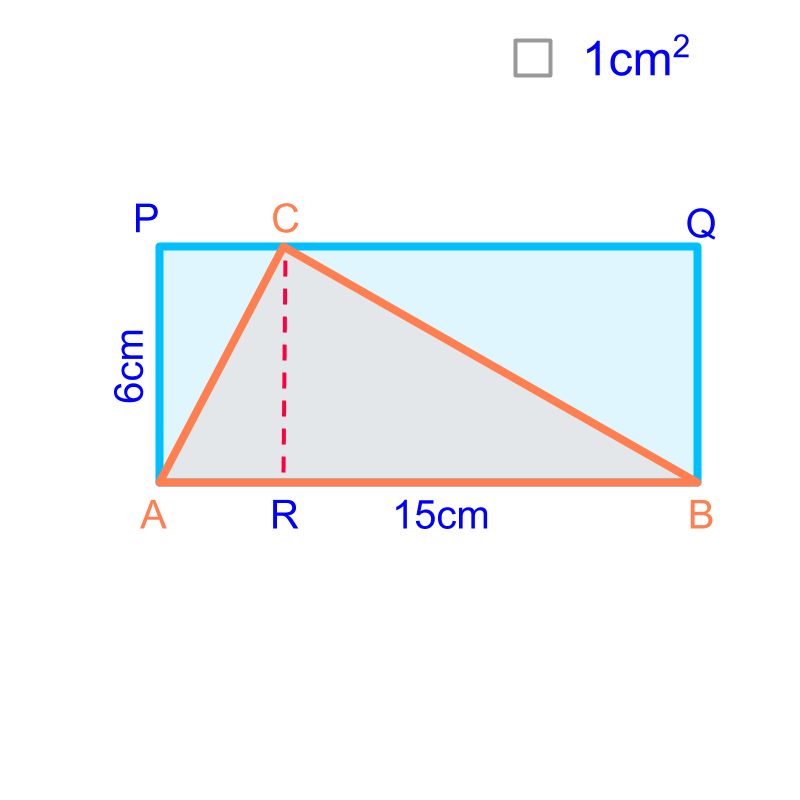The triangle of base $15$$15$ cm and height $6$$6$ cm is placed over a rectangle of length $15$$15$ cm and width $6$$6$ cm.

The rectangle is visualized into two parts $ARCP$$A R C P$ and $RBQC$$R B Q C$

The triangle is visualized into two parts $△ARC$$\triangle A R C$ and $△RBC$$\triangle R B C$

It is noted that the area of the triangle $△ARC$$\triangle A R C$ is half of the rectangle $ARCP$$A R C P$ and area of the triangle $△RBC$$\triangle R B C$ is half of the rectangle $RBQC$$R B Q C$.

The area of the triangle $△ABC$$\triangle A B C$ is sum of area of the two triangles $△ARC$$\triangle A R C$ and $△CRB$$\triangle C R B$.

$=$$=$ half the area of rectangles $ARCP$$A R C P$ and $CRBQ$$C R B Q$.

$=\frac{1}{2}×\overline{AR}×\overline{RC}+\frac{1}{2}×\overline{RB}×\overline{RC}$$= \frac{1}{2} \times \overline{A R} \times \overline{R C} + \frac{1}{2} \times \overline{R B} \times \overline{R C}$

$=\frac{1}{2}×\overline{RC}×\left(\overline{AR}+\overline{RB}\right)$$= \frac{1}{2} \times \overline{R C} \times \left(\overline{A R} + \overline{R B}\right)$

$=\frac{1}{2}×\overline{RC}×\overline{AB}\right)$

$=\frac{1}{2}×\phantom{\rule{1ex}{0ex}}\text{base}\phantom{\rule{1ex}{0ex}}×\phantom{\rule{1ex}{0ex}}\text{height}$$= \frac{1}{2} \times \textrm{b a s e} \times \textrm{h e i g h t}$

The area of the triangle is half of the area of rectangle OR in other words, $\frac{1}{2}×\phantom{\rule{1ex}{0ex}}\text{base}\phantom{\rule{1ex}{0ex}}×\left(height\right)$$\frac{1}{2} \times \textrm{b a s e} \times \left(h e i g h t\right)$

type 3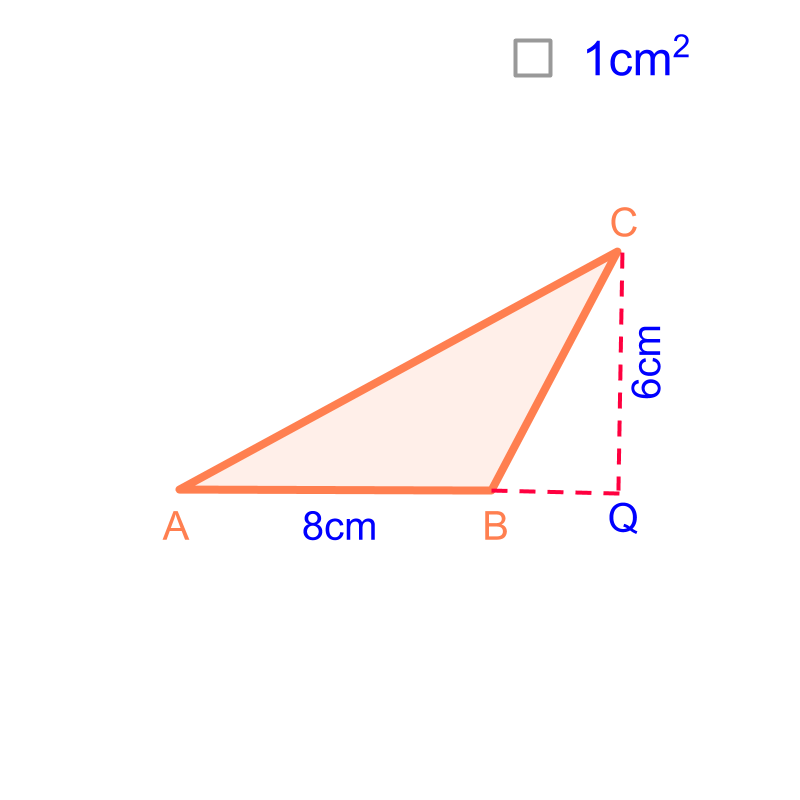Consider the triangle given in the figure. The base is $8$$8$ cm and height is $6$$6$ cm. To calculate the area, compare the triangle with a rectangle covering $AQC$$A Q C$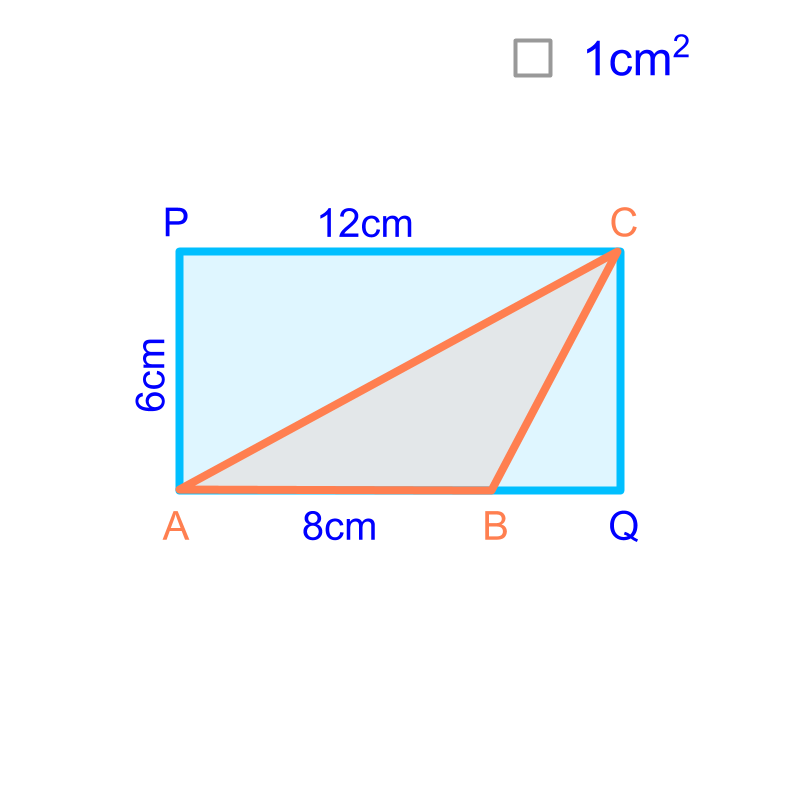The triangle of base $8$$8$ cm and height $6$$6$ cm is placed over a rectangle $AQCP$$A Q C P$. The rectangle is visualized to two triangles $△AQC$$\triangle A Q C$ and $\angle APC$$\angle A P C$. The area of triangle $△AQC$$\triangle A Q C$ is half of the rectangle.

The area of the triangle $△ABC$$\triangle A B C$ is

$=$$=$ area of $△AQC-$$\triangle A Q C -$ area of $△BQC$$\triangle B Q C$

$=\frac{1}{2}×\overline{AQ}×\overline{QC}-$$= \frac{1}{2} \times \overline{A Q} \times \overline{Q C} -$ $\frac{1}{2}×\overline{BQ}×\overline{QC}$$\frac{1}{2} \times \overline{B Q} \times \overline{Q C}$

$=\frac{1}{2}×\overline{QC}×\left(\overline{AQ}-\overline{BQ}\right)$$= \frac{1}{2} \times \overline{Q C} \times \left(\overline{A Q} - \overline{B Q}\right)$

$=\frac{1}{2}×\overline{QC}×\overline{AB}$$= \frac{1}{2} \times \overline{Q C} \times \overline{A B}$

$=\frac{1}{2}×\phantom{\rule{1ex}{0ex}}\text{base}\phantom{\rule{1ex}{0ex}}×\phantom{\rule{1ex}{0ex}}\text{height}$$= \frac{1}{2} \times \textrm{b a s e} \times \textrm{h e i g h t}$

The area of the triangle is half of the area of rectangle OR in other words, $\frac{1}{2}×\phantom{\rule{1ex}{0ex}}\text{base}\phantom{\rule{1ex}{0ex}}×\left(height\right)$$\frac{1}{2} \times \textrm{b a s e} \times \left(h e i g h t\right)$

common formula

Consider the three types of triangles,For all the types, the area is derived as

$\frac{1}{2}×\phantom{\rule{1ex}{0ex}}\text{base}\phantom{\rule{1ex}{0ex}}×\left(height\right)$$\frac{1}{2} \times \textrm{b a s e} \times \left(h e i g h t\right)$

What is the area of the triangle with base $2$$2$cm and height $3$$3$cm?
The answer is "$\frac{1}{2}×2×3=3c{m}^{2}$$\frac{1}{2} \times 2 \times 3 = 3 c {m}^{2}$"

summary

Area of a Triangle:
$\frac{1}{2}×\phantom{\rule{1ex}{0ex}}\text{base}\phantom{\rule{1ex}{0ex}}×\left(height\right)$$\frac{1}{2} \times \textrm{b a s e} \times \left(h e i g h t\right)$Outline

The outline of material to learn "Mensuration basics : Length, Area, & Volume" is as follows.

•  Measuring Basics

→   Introduction to Standards

→   Measuring Length

→   Accurate & Approximate Meaures

→   Measuring Area

→   Measuring Volume

→   Conversion between Units of Measure

•  2D shapes

→   Perimeter of Polygons

→   Area of Square & rectangle

→   Area of Triangle

→   Area of Polygons

→   Perimeter and area of a Circle

→   Perimeter & Area of Quadrilaterals

•  3D shapes

→   Surface Area of Cube, Cuboid, Cylinder

→   Volume of Cube, Cuboid, Cylinder